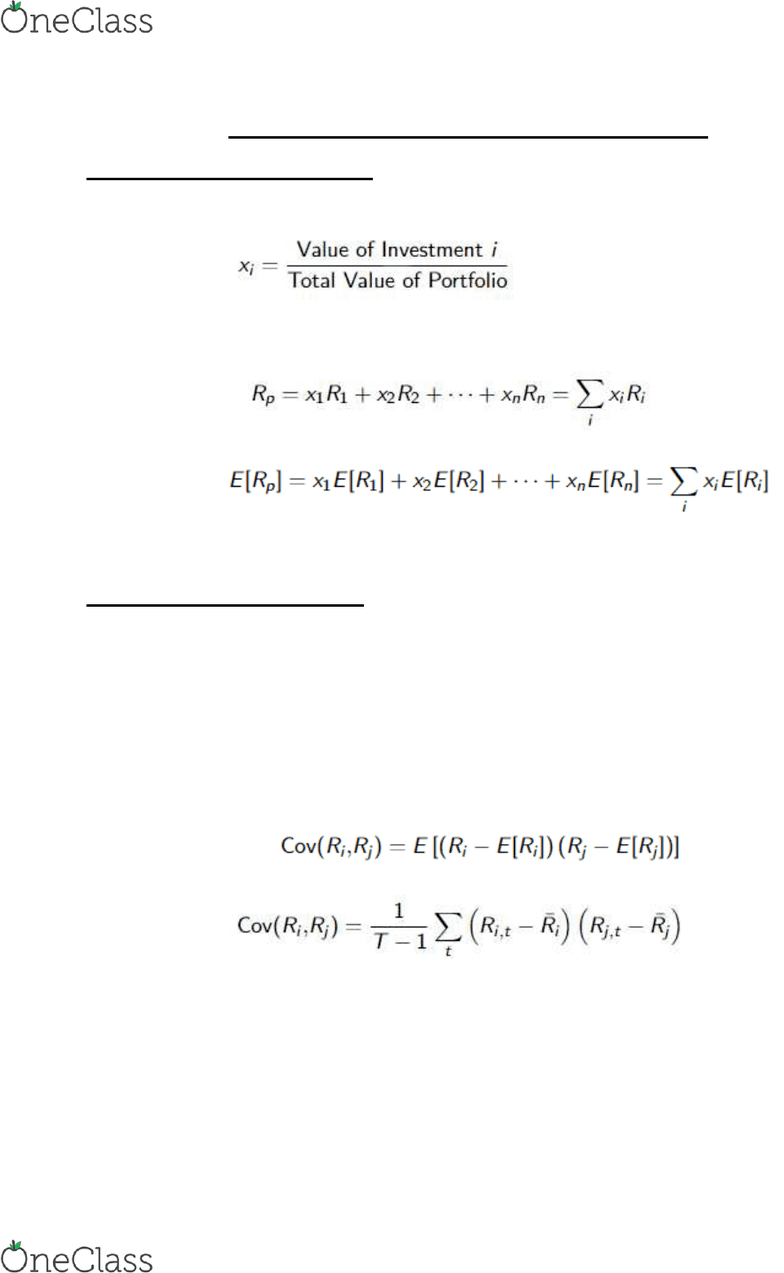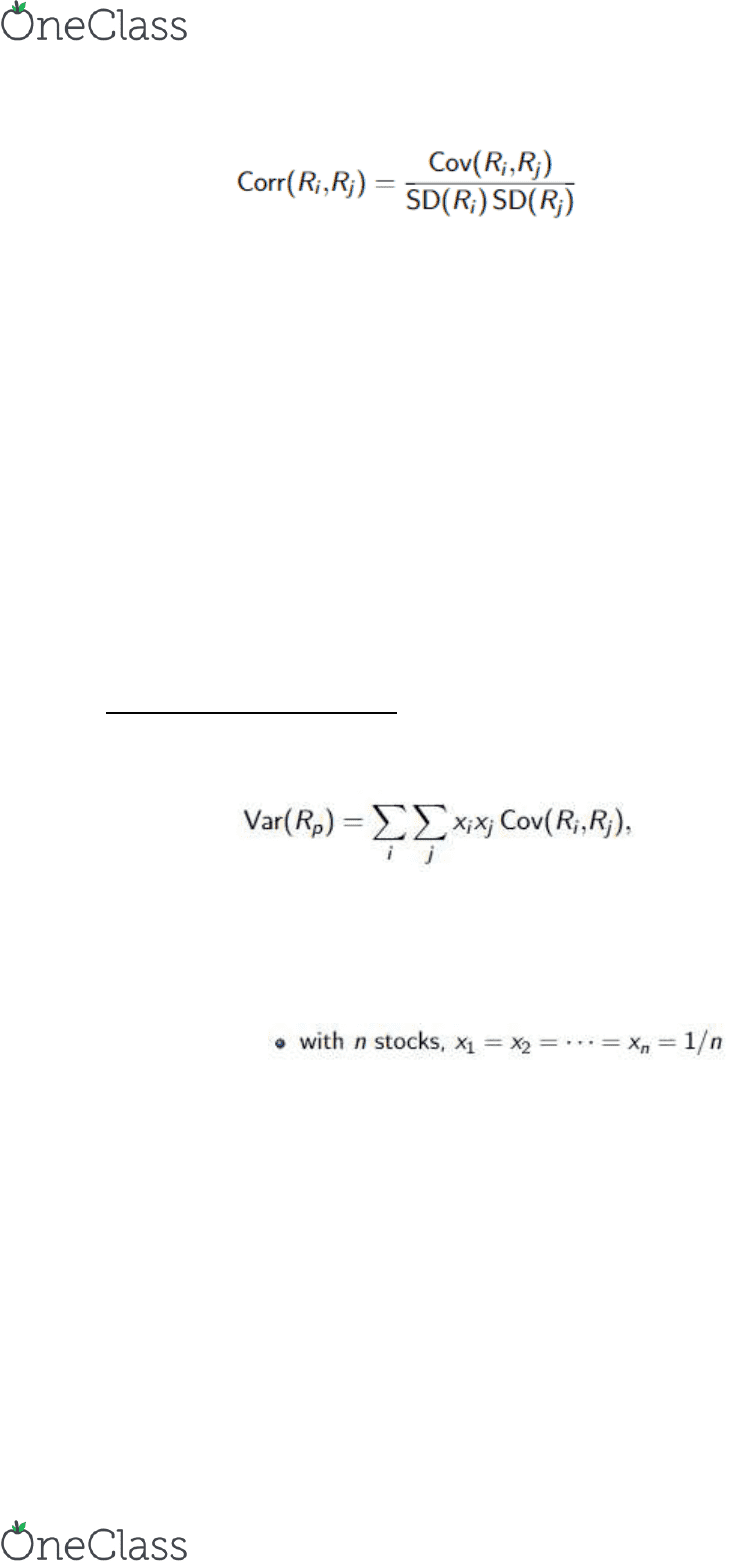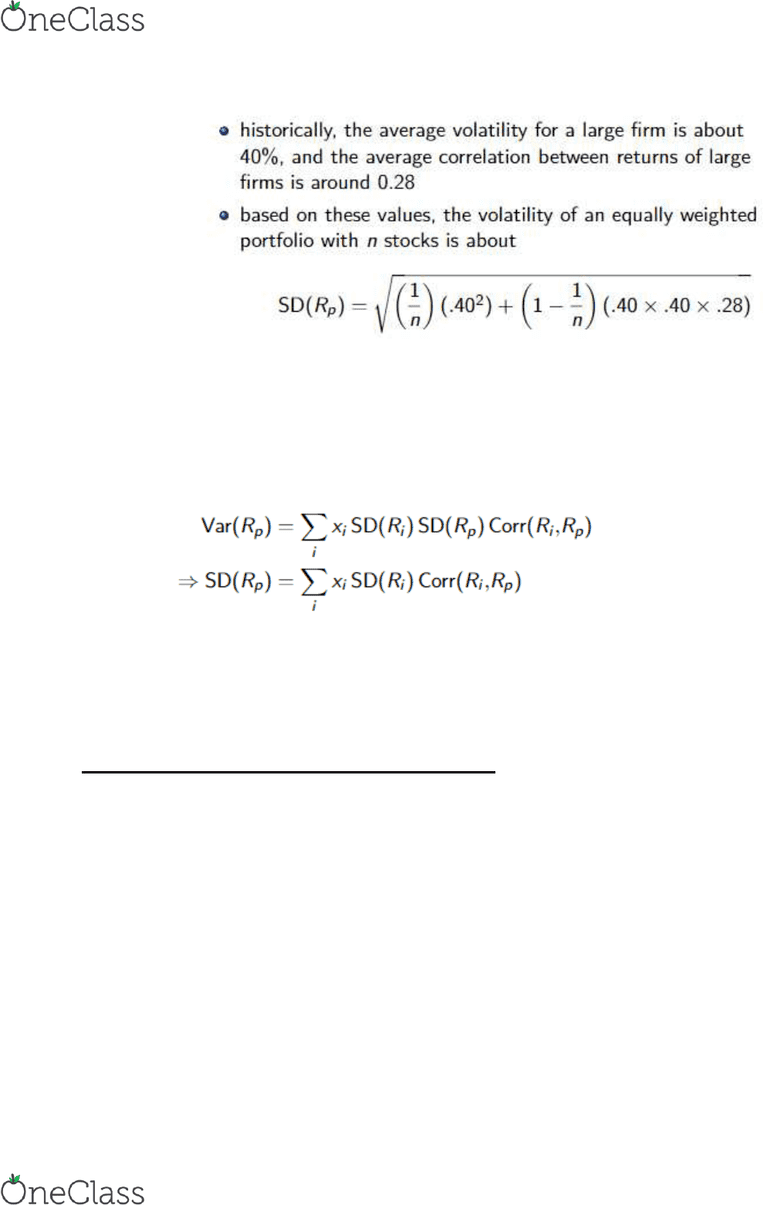# AFM273 Chapter Notes - Chapter 11: Weighted Arithmetic Mean, Expected Return, Covariance

64 views8 pages1
C11: Optimal portfolio choice and the CAPM
The expected return of a portfolio
We can describe a portfolio by its portfolio weights
o How much of each investment makes up the portfolio
Portfolio weights add up to 1
o If xi is the weighted average of portfolio, then Ri would be the weighted average of the
realized returns
o So the expected returns would be
Note: without trading, the weights will increase for stocks in the portfolio whose returns
exceed the portfolios
Volatility of a two stock portfolio
Combining risks
o By combining stocks into a portfolio we can diversify risk
This allows risk in a portfolio to be lower than individual stocks
o Amount of risk that is eliminated in a portfolio depends on the sensitivity of the stock's
price to common risk
If everything in the portfolio is related to a single sector, minimal risk is eliminated
If there is a great degree of diversification then more risk can be eliminated
Determining covariance and correlation
o Covariance
Expected product of the deviations of2 returns from their means
o Formula for estimating covariance from historical data
o If stocks move together, returns will tend to be above or below average at the same
time
Covariance is positive
o If stocks move opposite
Covariance is negative
o Magnitude of covariance is hard to interpret
Correlation
o Value of correlation will be larger the more volatile
Also be larger if it moves more closely relative to other stocks
find more resources at oneclass.com
find more resources at oneclass.com
Unlock document

This preview shows pages 1-3 of the document.
Unlock all 8 pages and 3 million more documents.2
o
Measures the strength of the relationship between returns while controlling the
stock volatilities
Value output is always between -1 and 1
Closer to -1, perfect negative correlation (moves opposite)
Closer to 1, perfect positive correlation (moves tandem)
When equals 0 no correlation
o Stock returns usually move together if they have they are affected by the same
economic events
Stocks in the same industry usually affected by the same things and may move
together
Computing a portfolio's variance and volatility
o The variance of a portfolio is equal to the weighted average covariance for each stock
with the portfolio
Variance of 2 stock portfolio
Var(Rp)=X12Var(R1)+X22Var(R2)+2X1X2Cov(R1,R2)
Standard deviation
SD(Rp) = Sqrt Var(Rp)
Volatility of a large portfolio
Large portfolio variance
o The variance of a portfolio is equal to the weighted average covariance for each stock
with the portfolio
o
o The risk of a portfolio depends on how each stock's return moves in relation to it
The variability of a portfolio depends on the co-movement of the stocks
Variance of a portfolio is the sum of all the covariance's of the returns of all pairs
of stocks in the portfolio multiplied by each of their portfolio weights
Diversification with an equally weighted portfolio
o Equally weighted portfolio is when each stock has the same amount invested in it
o Variance of an equally weighted portfolio of n Stocks
Var(Rp)=1/n(average variance of the individual stocks) + (1-1/n)(average
covariance between the stocks)
o Variance of a large portfolio mainly determined by the average covariance among the
stocks
find more resources at oneclass.com
find more resources at oneclass.com
Unlock document

This preview shows pages 1-3 of the document.
Unlock all 8 pages and 3 million more documents.3
With the formula above, as the portfolio gets larger and (n) increases,
First portion will approach 0, while the second portion approaches 1
The standard deviation would then be the square root of the average
covariance between the stocks
o Effects of diversification are largest at the initial change
Effects can be observed with a few stocks
Still must carefully choose stocks that are not correlated to be well diversified
Diversification with general portfolios
o
o Each security contributes to the volatility of the portfolio according to its total risk scaled
by its correlation to the portfolio
The correlation to the portfolio adds a fraction from the risk of the stock to the
portfolio
o Unless all stocks are perfectly correlated, the risk of the portfolio will be lower than the
weighted average volatility of the individual stocks
Risk Versus Return: Choosing an efficient portfolio
Efficient portfolio with two stocks
o Different weights will vary the volatility and expected return
o These varying weights can be turned into a graph
Inefficient portfolios
o Occur when there can be another portfolio that is better in expected returns/ less
volatility
Moving northwest
Efficient portfolios
o No other portfolio of the 2 stocks that will offer less volatility for a higher return
Efficient portfolios are different to people depending how risk averse they may
be
The effect of correlation
o Correlation doesn’t effect on the expected return of a portfolio
o Correlation does affect that volatility
As correlation decreases larger effects to volatility since diversification starts
making a large impact
o No diversification, points connected by a straight line
find more resources at oneclass.com
find more resources at oneclass.com
Unlock document

This preview shows pages 1-3 of the document.
Unlock all 8 pages and 3 million more documents.